International
Tables for
Crystallography
Volume F
Crystallography of biological macromolecules
Edited by M. G. Rossmann and E. Arnold

International Tables for Crystallography (2006). Vol. F, ch. 2.1, pp. 47-52   | 1 | 2 |

Section 2.1.3. Point groups and crystal systems

J. Drentha*

aLaboratory of Biophysical Chemistry, University of Groningen, Nijenborgh 4, 9747 AG Groningen, The Netherlands
Correspondence e-mail: j.drenth@chem.rug.nl

2.1.3. Point groups and crystal systems

| top | pdf |

If symmetry can be recognised in the external shape of a body, like a crystal or a virus molecule, corresponding symmetry elements have no translations, because internal translations (if they exist) do not show up in macroscopic properties. Moreover, they pass through one point, and this point is not affected by the symmetry operations (point-group symmetry). For idealized crystal shapes, the symmetry axes are limited to one-, two-, three-, four- and sixfold rotation axes because of the space-filling requirement for crystals. With the addition of mirror planes and inversion centres, there are a total of 32 possible crystallographic point groups.

Not all combinations of axes are allowed. For instance, a combination of two twofold axes at an arbitrary angle with respect to each other would multiply to an infinite number of twofold axes. A twofold axis can only be combined with another twofold axis at. A third twofold axis is then automatically produced perpendicular to the first two (point group 222). In the same way, a threefold axis can only be combined with three twofold axes perpendicular to the threefold axis (point group 32).

For crystals of biological macromolecules, point groups with mirrors or inversion centres are not allowed, because these molecules are chiral. This restricts the number of crystallographic point groups for biological macromolecules to 11; these are the enantiomorphic point groups and are presented in Table 2.1.3.1.Table 2.1.3.1| top | pdf | The 11 enantiomorphic point groups
 The point groups are presented as two stereographic projections (see Fig. 2.1.3.1).On the right is a projection of the symmetry elements, and on the left a projection of the general faces. They are arranged according to the crystal system to which they belong: triclinic, monoclinic etc. Different point groups are separated by full horizontal rules. The monoclinic point groups are given in two settings: in the conventional setting with the twofold axis along b (unique axis b), and the other setting with unique axis c. The b axis is horizontal in the projection plane, and the c axis is normal to the plane. Three-, four- and sixfold axes are always set along the c axis, normal to the plane. A special case is the trigonal system; either hexagonal axes or rhombohedral axes can be chosen. In the hexagonal case, the threefold axis is along the c axis. The other two axes are chosen along or between the twofold axes, which include an angle of. In the rhombohedral setting, the threefold axis is along the body diagonal of the unit cell, and the unit cell vectors a, b and c are the shortest non-coplanar lattice vectors symmetrically equivalent with respect to the threefold axis (Fig. 2.1.3.2). Symbols: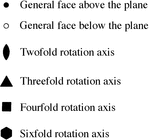Adapted with permission from IT A (2005), Table 10.1.2.2.
 TRICLINIC 1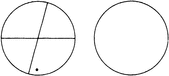MONOCLINIC 2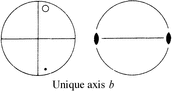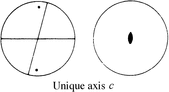ORTHORHOMBIC 222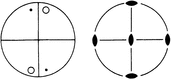TETRAGONAL 4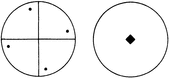TETRAGONAL 422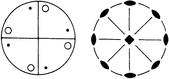TRIGONAL Hexagonal axes 3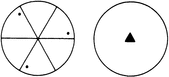TRIGONAL Rhombohedral axes 3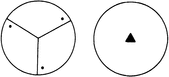TRIGONAL Hexagonal axes 321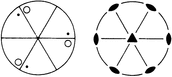TRIGONAL Hexagonal axes 312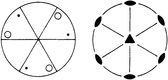TRIGONAL Rhombohedral axes 32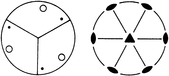HEXAGONAL 6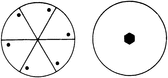HEXAGONAL 622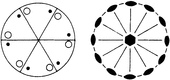CUBIC 23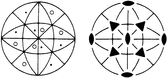CUBIC 432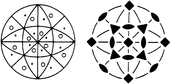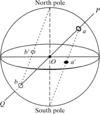Figure 2.1.3.1 | top | pdf |How to construct a stereographic projection. Imagine a sphere around the crystal with O as the centre. O is also the origin of the coordinate system of the crystal. Symmetry elements of the point groups pass through O. Line OP is normal to a crystal plane. It cuts through the sphere at point a. This point a is projected onto the horizontal plane through O in the following way: a vertical dashed line is drawn through O normal to the projection plane and connecting a north and a south pole. Point a is connected to the pole on the other side of the projection plane, the south pole, and is projected onto the horizontal plane at. For a normal OQ intersecting the lower part of the sphere, the point of intersection b is connected to the north pole and projected at. For the symmetry elements, their points of intersection with the sphere are projected onto the horizontal plane.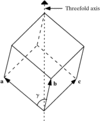Figure 2.1.3.2 | top | pdf |A rhombohedral unit cell.

Although the crystals of asymmetric molecules can only belong to one of the 11 enantiomorphic point groups, it is nevertheless important to be aware of the other point groups, especially the 11 centrosymmetric ones (Table 2.1.3.2).This is because if anomalous scattering can be neglected, the X-ray diffraction pattern of a crystal is always centrosymmetric, even if the crystal itself is asymmetric (see Sections 2.1.7and 2.1.8).

 Table 2.1.3.2| top | pdf | The 11 point groups with a centre of symmetry
 For details see Table 2.1.3.1.Projections of mirror planes are indicated by a bold line or circle. The inversion centre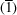is indicated by a small circle at the origin. Adapted with permission from IT A (2005), Table 10.1.2.2.
 TRICLINIC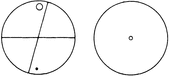MONOCLINIC 2/m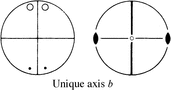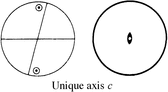ORTHORHOMBIC mmm or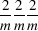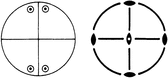TETRAGONAL 4/m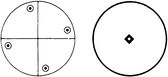TETRAGONAL 4/mmm or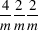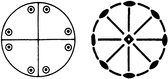TRIGONAL Hexagonal axes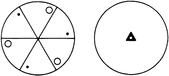TRIGONAL Rhombohedral axes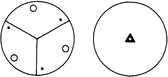TRIGONAL Hexagonal axesor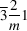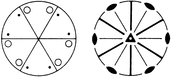TRIGONAL Hexagonal axesor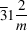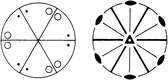TRIGONAL Rhombohedral axesor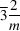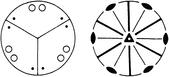HEXAGONAL 6/m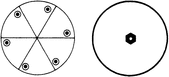HEXAGONAL 6/mmm or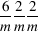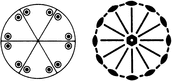CUBICor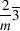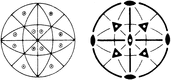CUBICor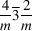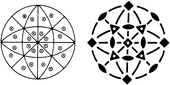The protein capsids of spherical virus molecules have their subunits packed in a sphere with icosahedral symmetry (532). This is the symmetry of a noncrystallographic point group (Table 2.1.3.3).A fivefold axis is allowed because translation symmetry does not apply to a virus molecule. Application of the 532 symmetry leads to 60 identical subunits in the sphere. This is the simplest type of spherical virus (triangulation number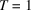). Larger numbers of subunits can also be incorporated in this icosahedral surface lattice, but then the subunits lie in quasi-equivalent environments and T assumes values of 3, 4 or 7. For instance, for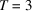particles there are 180 identical subunits in quasi-identical environments.

 Table 2.1.3.3| top | pdf | The icosahedral point group 532
 For details see Table 2.1.3.1.Adapted with permission from IT A (2005), Table 10.1.4.3.
 ICOSAHEDRAL 532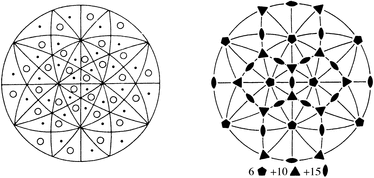On the basis of their symmetry, the point groups are subdivided into crystal systems as follows. For each of the point groups, a set of axes can be chosen displaying the external symmetry of the crystal as clearly as possible, and, in this way, the seven crystal systems of Table 2.1.3.4are obtained. If no other symmetry is present apart from translational symmetry, the crystal belongs to the triclinic system. With one twofold axis or screw axis, it is monoclinic. The convention in the monoclinic system is to choose the b axis along the twofold axis. The orthorhombic system has three mutually perpendicular twofold (screw) axes. Another convention is that in tetragonal, trigonal and hexagonal crystals, the axis of highest symmetry is labelled c. These conventions can deviate from the guide rules for unit-cell choice given in Section 2.1.1.Table 2.1.3.4| top | pdf | The seven crystal systems
Crystal systemConditions imposed on cell geometryMinimum point-group symmetry
Triclinic None 1
Monoclinic Unique axis b:2
Orthorhombic222
Tetragonal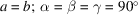4
Trigonal Hexagonal axes: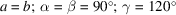3
Rhombohedral axes: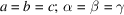Hexagonal6
Cubic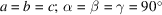23
A rhombohedral unit cell can be regarded as a cube extended or compressed along the body diagonal (the threefold axis) (see Fig. 2.1.3.2).

The seven crystal systems are based on the point-group symmetry. Except for the triclinic unit cell, all other cells can occur either as primitive unit cells or as centred unit cells (Section 2.1.1). A total of 14 different types of unit cell exist, depicted in Fig. 2.1.3.3.Their corresponding crystal lattices are commonly called Bravais lattices.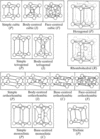Figure 2.1.3.3 | top | pdf |The 14 Bravais lattices. Reproduced with permission from Burzlaff & Zimmermann (2005).